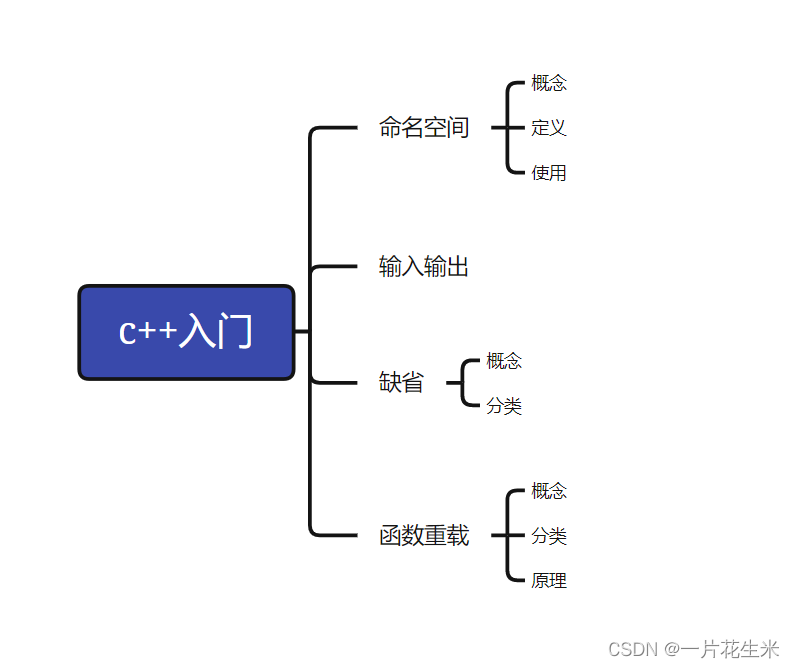0

# c++入门（命名空间，输入输出，缺省，函数重载）## 定义

namespace关键字+命名空间名字+{}，{}中为命名空间的成员

``````namespace abc
{int a =10;}
``````

1.命名空间可以定义变量/函数/类型

``````namespace bite
{int a =10;//定义变量intAdd(int left,int right)//定义函数{return left + right;}structNode//定义类型{structNode* next;int x;};}
``````

2.命名空间可以嵌套定义

``````namespace Node1
{int a =10;namespace Node2
{int b =20;}}
``````

3.同一个工程可以允许存在名称相同的多个命名空间，编译器最后会将名称一样的命名空间进行合并（同级进行合并）

``````//text。cppnamespace Node1
{int a;}//text.hnamespace Node1
{int b;}//此时两个文件里面同名的命名空间Node1会被合并成一个
``````

1.在命名空间中定义的变量都属于全局变量，存在静态区

2.命名空间不会影响变量的生命周期，只是限定域，只影响编译器查找规则
3.编译器的查找规则是：先局部查找，再全局查找，如果指定了命名空间就在命名空间上找，找不到就会报错
4.预处理阶段之后头文件就会被展开，存放在全局之中；也就是.h不会被处理
5.同一个域里面也不能出现命名冲突问题，就是说在一个命名空间里面也不能出现变量名相同的情况

## 使用

（三种使用方式）
1.加命名空间名称及作用域限定符(指定访问)

``````intmain(){printf("%d\n",Node1::a);return0;}
``````

2.使用using namespace命名空间名称 引入（全部展开）

``````usingnamespace Node1;intmain(){printf("%d\n",Node1::a);printf("%d\n",b);return0;}
``````

using namespace std 将c++标准库里的东西存入的命名空间std展开来

3.使用using将命名空间的成员挨个引入（部分展开）

``````using Node1::a;intmain(){printf("%d\n",Node1::a);return0;}
``````

## 输入输出

``````#include<iostream>usingnamespace std;intmain(){
cout <<"Hello world!"<< endl;return0;}
``````

1.<<是流插入运算符，>>是流提取运算符，cout和cin是全局流对象，endl是换行符号，都包含在iostream，<<与cout一起使用输出，>>与cin一起使用输入
2.使用cout标准输出对象（控制台）和cin标准输入对象（键盘）时，必须包含iostream头文件以及用以上三种方法调用std
3.c++的输入输出特性比较c语言的就是可以自动识别变量类型

## 缺省参数概念

``````voidFunc(int a =0){
cout << a << endl;}intmain(){Func();//没有传参，使用缺省Func(10);//有传参，使用传参值return0;}
``````

## 分类

1.全缺省参数

``````voidFunc(int a =10,int b =20,int c =30){
cout<<"a ="<<a<<endl;
cout<<"b ="<<b<<endl;
cout<<"c ="<<c<<endl;}
``````

2.半缺省参数

``````voidFunc(int a,int b =20,int c =30){
cout<<"a ="<<a<<endl;
cout<<"b ="<<b<<endl;
cout<<"c ="<<c<<endl;}
``````

1.半缺省参数传参必须传一个，必须从右往左依次传参，不能间隔
2.缺省参数不能在函数声明和定义中同时出现（防止出现矛盾，默认参数重定义问题）

## 概念

c++允许在同一作用域中声明几个功能类似的同名函数，这些函数的形参列表（参数个数，类型，类型顺序）不同，常用来处理实现功能类似数据类型不同的问题。

## 分类

1.参数类型不同

``````intAdd(int left,int right){
cout<<"int Add(int left,int right)"<< endl;return left+right;}doubleAdd(double left,double right){
cout<<"double Add(double left,double right)"<<endl;return left+right;}
``````

2.参数个数不同

``````voidf(){
cout<<"f()"<< endl;}voidf(int a){
cout<<"f(int a)"<< endl;}
``````

3.参数类型顺序不同

``````voidf(int a,char b){
cout<<"f(int a,char b)"<< endl;}voidf(char b,int a){
cout<<"f(char b,int a)"<< endl;}
``````

``````voidf(){
cout<<"f()"<<endl;}voidf(int a =0,char b =1){
cout<<"f(int a = 0, char b = 1)"<<endl;}intmain(){f(10);f(10,20);f();return0;}
``````

f构成函数重载，但是f()有二义性（歧义），因此会报错

## 原理

c++支持函数重载的原因是因为有名字修饰
1.函数重载是由于参数的个数，顺序，类型不同实现，是因为只要参数不同，修饰出来的名字就不一样，由此支持了函数重载
2.c语言不支持函数重载是因为同名函数无法区分
3.如果两个函数参数和函数名一样，只有返回值不同是不会构成重载的，因为调用的时候编译器无法区分使用返回值是什么类型的函数，出现二义性

### “c++入门（命名空间，输入输出，缺省，函数重载）”的评论:

##### 关于作者##### overfit同步小助手